×#### Thank you for registering.

One of our academic counsellors will contact you within 1 working day.

Click to Chat

1800-1023-196

+91-120-4616500

CART 0

• 0

MY CART (5)

Use Coupon: CART20 and get 20% off on all online Study Material

ITEM
DETAILS
MRP
DISCOUNT
FINAL PRICE
Total Price: Rs.

There are no items in this cart.
Continue Shopping• Complete JEE Main/Advanced Course and Test Series
• OFFERED PRICE: Rs. 15,900
• View Details

```Revision Notes on Exponents and Powers

Introduction to Exponents and Powers

When the numbers given are very large like 54,32,00,00,000 then it is not easy to read them so we write them in the form of exponents.

Exponents make these numbers easy to read, write, understand and compare.

Exponents

To write the large numbers in short form, we use exponents.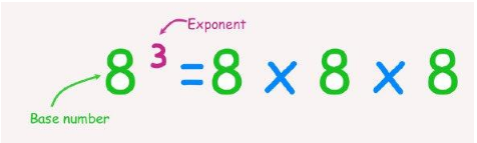Here 8 is the base, 3 is the exponent and 83 is the exponential form of 512.

This can be read as “8 raised to the power of 3”.

The Expanded form of Natural Numbers

When we write the expanded form of a natural number then it can be written in exponential form.

Example

247983 = 2 × 100000 + 4 × 10000 + 7 × 1000 + 9 × 100 + 8 × 10 + 3 × 1

= 2 × 105 + 4 × 104 + 7 × 103 + 9 × 102 + 8 × 101 + 3 × 1

Some Important Points to Remember

(-1)odd number = (-1)

(-1)even number = (1)

a3b2 ≠ a2b3

a2b3 = b3a2

Laws of Exponents

1. How to multiply powers with the same base?

If we have to multiply the powers which have the same base then we have to add the exponents.

am × an = am + n

Example

83 × 84 = 83 + 4 = 87

2. How to divide powers with the same base?

If we have to divide the powers which have the same base then we have to subtract the exponents.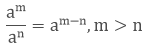Example3. How to take the power of a power?

If we have to take the power of a power then we have to multiply the exponents.

(am)n = amn

Example

(83)4 = 83 × 4 = 812

4. How to multiply the powers with the same exponents?

If we have to multiply the powers where the base is different but exponents are same then we will multiply the base.

ambm = (ab)m

Example

8343 = (8× 4)3 = 323

5. How to divide the powers with the same exponents?

If we have to divide the powers where the base is different but exponents are same then we will divide the base.Example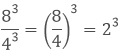6. Numbers with Exponent Zero

Any number with zero exponents is equal to one irrespective of the base.

a° = 1

Example

8° = 1

7. Numbers with Exponent One

Any number with one as the exponent is equal to the number itself.

a1 = a

Example

81 = 8

8. Power with a Negative Exponent

Negative exponents can be converted into positive exponents.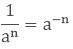Example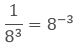Miscellaneous Examples

Example: 1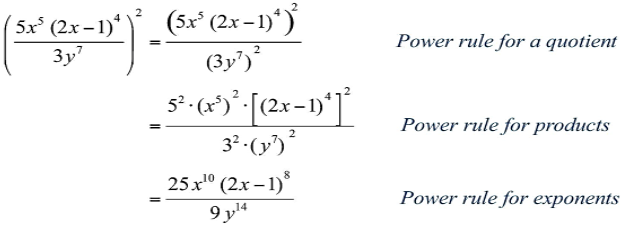Example: 2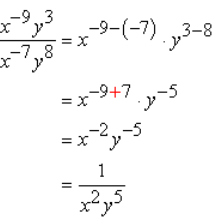Expressing Large Numbers in the Standard Form

If we have to write very large numbers then to make them easy to read and understand we can write them in the standard form using decimals and exponents from 1.0 to 10.0.

85 = 8.5 × 10 = 8.5 × 101

850 = 8.5 × 100 = 8.5 × 102

8500 = 8.5 × 1000 = 8.5 × 103

8500 = 8.5 × 10000 = 8.5 × 104

and so on.
```### Course Features

• 728 Video Lectures
• Revision Notes
• Previous Year Papers
• Mind Map
• Study Planner
• NCERT Solutions
• Discussion Forum
• Test paper with Video Solution